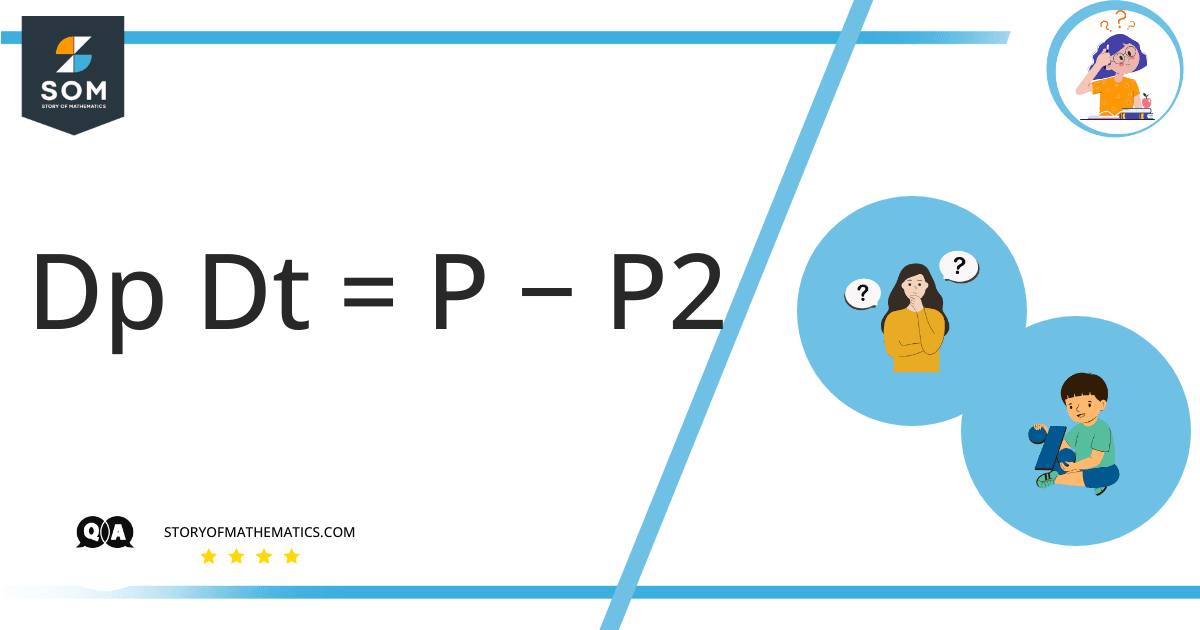# Solve the differential equation dp/dt=p−p^2In this question, we have to find the Integration of the given function $\dfrac{dP}{dt}= \left[P – P^{2} \right]$ by rearranging the equation.

The basic concept behind this question is the knowledge of derivatives, integration, and the rules such as the product and quotient rules of integration.

Given function:

$\dfrac{dP}{dt}= \left[P – P^{2} \right]$

First, we will rearrange the given equation with $P$ on one side of the equation and $t$ on the other. For this, we have the following equation:

$dP = \left[P – P^{2} \right] {dt}$

$\dfrac{1 }{\left[P – P^{2} \right]} dP = dt$

$dt =\dfrac{1 }{\left[P – P^{2} \right]} dP$

Take Integration on both sides of the equation. We get:

$\int dt = \int \dfrac{1 }{P – P^{2}} dP$

Taking $P$ common on the right-hand side, we will have the equation:

$\int dt = \int \dfrac{1 }{P (1 – P)} dP$

As we can write $1 = ( 1-P ) + P$ in the above equation, putting it in the question we have the following equation:

$\int dt = \int \dfrac{(1-P) + P }{P (1 – P)} dP$

$\int dt = \int \dfrac{(1-P) }{P (1 – P)} dP + \int \dfrac{P }{P (1 – P)} dP$

Canceling $1-P$ from the denominator and numerator of the equation:

$\int dt = \int \dfrac{1 }{P } dP + \int \dfrac{P }{P (1 – P)} dP$

Canceling $P$ from the denominator and numerator of the equation:

$\int dt = \int \dfrac{1 }{P } dP + \int \dfrac{1 }{ (1 – P)} dP$

Solving the above equation now:

$t + c_1 = \ln{\left|P \right|\ -\ }\ln{\left|1-P\right|\ }$

$t + c_1 =\ln{\left|\ \frac{ P }{ 1 – P }\ \right|}$

$e^{ t + c_{1} } =e^{\ln{\left|\ \dfrac{ P }{ 1 – P }\ \right|}}$

We know that $e^{\ln{x} } = x$ so we have the above equation as:

$e^{ t} e^{ c_1 } = \left| \dfrac { P }{ 1 – P } \right|$

$\left| \dfrac { P }{ 1-P } \right| = e^{ t} e^{ c_1 }$

$\dfrac { P }{ 1-P } = \pm e^{ t} e^{ c_1 }$

Let us suppose that another constant $c$ is introduced in the equation which is $\pm e^{ c_1 } = c$. Now the equation becomes:

$\dfrac { P }{ 1-P } = ce^{ t}$

Multiplying by $1-P$ on both the side of the equation:

$P=c e^t (1-P)$

$P = ce^t- ce^{t}P$

$P+ ce^{t}P = ce^t$

$P(1+ ce^{t}) = ce^t$

$P= \dfrac{ce^t}{(1+ ce^{t})}$

## Numerical Result

$P= \dfrac{ce^t}{(1+ ce^{t})}$

## Example

Integrate the equation:

$\int dt= \int \dfrac{1}{x } dx$

Solving the above equation now:

$t+c_1 = \ln{\left|x \right|}$

$e^{t+ c_1}=e^{\ln{x}}$

We know that $e^{\ln{x}} = x$ so we have the above equation as:

$e^{t} e^{ c_1}=x$